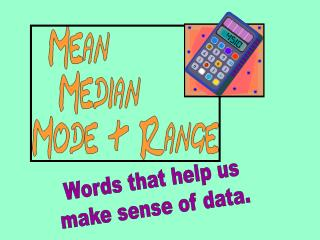DownloadDownload PresentationWords that help us make sense of data.

# Words that help us make sense of data.

Download Presentation## Words that help us make sense of data.

- - - - - - - - - - - - - - - - - - - - - - - - - - - E N D - - - - - - - - - - - - - - - - - - - - - - - - - - -
##### Presentation Transcript

1. Words that help us make sense of data.

2. These are Abby’s science test scores. 86 84 97 73 88 63 97 95 100

3. What can you tell us about these numbers? 86 84 97 73 63 88 97 100 95

4. Let's define these words & learn how to find them.

5. What is the MEAN?How do we find it? The mean is the numerical average of the data set. The mean is found by adding all the values in the set, then dividing the sum by the number of values.

6. Lets find Abby’s MEAN science test score? 97 84 Add all the values. 88 100 95 63 Divide the sum by the number of values. 73 86 783 9 ÷ + 97 The mean is 87 783

7. What is the MEDIAN?How do we find it? The MEDIAN is the number that is in the middle of a set of data 1. Arrange the numbers in the set in order from least to greatest. 2. Then find the number that is in the middle.

8. Arrange values from least to greatest. 63 100 73 88 95 97 84 86 97 Find the number that is in the middle. The median is 88. Half the numbers are less than the median. Half the numbers are greater than the median.

9. Median Sounds like MEDIUM Think middlewhen you hear median. large medium small

10. How do we findthe MEDIAN when two numbers are in the middle? 1. Add the two numbers. 2. Then divide by 2.

11. Arrange values from least to greatest. 100 63 88 95 97 73 84 97 There are two numbers in the middle. Add the 2 numbers. Divide by 2. 88 + 95 = 183 183 ÷ 2 The median is 91.5

12. What is the MODE?How do we find it? The MODE is the piece of data that occurs most frequently in the data set. A set of data can have: • One mode • More than one mode • No mode

13. Arranging values from least to greatest makes it easier to find the mode. 63 100 73 88 95 97 84 86 97 Find the number that appears more or most frequently. The value 97 appears twice. All other numbers appear just once. 97 is the MODE

14. MODE A Hint for remembering the MODE… The first two letters give you a hint… MOde Most Often MODE MOST OFTEN

15. Which set of data has ONE MODE? 9, 11, 16, 6, 7, 17, 18 A B 18, 7, 10, 7, 18 C 9, 11, 16, 8, 16

16. Which set of data has NO MODE? 9, 11, 16, 6, 7, 17, 18 A B 18, 7, 10, 7, 18 C 13, 12, 12, 11, 12

17. Which set of data has MORE THAN ONE MODE? 9, 11, 16, 8, 16 A 9, 11, 16, 6, 7, 17, 18 B C 18, 7, 10, 7, 18

18. What is the RANGE?How do we find it? The RANGE is the difference between the lowest and highest values. largest number smallest number - RANGE

19. Arranging values from least to greatest makes it easier to find the RANGE. 97 63 95 97 73 86 88 84 Subtract the lowest value from the highest. • 97 • 63 • 34 34 is the RANGE or spread of this set of data

20. What is the RANGE of this set of data? 84 48 86 99 71 97 88

21. What is the RANGE of this set of data? 71 86 88 97 48 99 84 • 99 • 48 51

22. What is the RANGE of this set of data? 33 48 46 17 67 15 85

23. What is the RANGE of this set of data? 46 15 17 33 85 48 67 • 85 • 15 70

24. What is the RANGE of this set of data? 267 119 357 329 401 227 483

25. What is the RANGE of this set of data? 267 357 401 119 227 329 483 • 483 • 119 364

26. Mean Median Mode This one is the requires more work than the others. Right in the MIDDLE. This one is the easiest to find— Just LOOK.

27. Mean Median Mode Find the….

28. Mean Median Mode

29. Mean Median Mode Find the….

30. Mean Median Mode

31. Mean Median Mode Find the…. & Range

32. Mean Median Mode Range

33. Mean Median Mode Find the…. & Range

34. Mean Median Mode Range

35. Mean Median Mode Find the…. & Range

36. Mean Median Mode Range